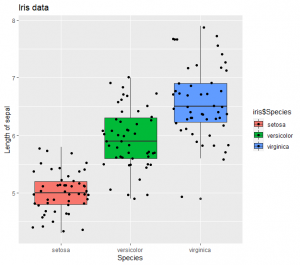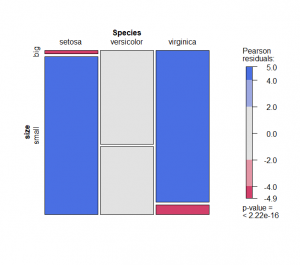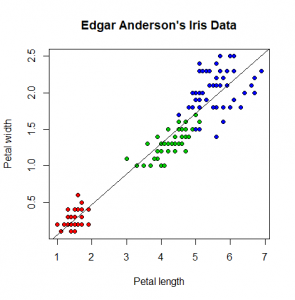# Basic statistical analysis with R

Asst. Prof. Teerasak E-kobon

29 Jan 2021

This chapter will guide how to basically use R programming in the statistical analysis which is an important skill for bioinformaticists and bioscientists to confidently state the research results to others. First, there are several data types to be mentioned including continuous data (infinite number), discrete data (integer), categorical data (specific set of values such as animal species), binary data (0 and 1), ordinal data (the ordinal data with level or rank), and unstructured data (text or string).

In R environment, the installed R language will come together with several freely available datasets for practical usage of the packages and libraries. One example is the iris dataset which can be simply called using the name, iris. When you try the head(iris) function, you will see the first few rows of this iris data table. The object is called the data frame in R. The symbol \$ is a special character used to select a specific column of the data frame table.

Example 1 Basic statistical functions in the R base package

nrow(iris)
ncol(iris)

names(iris)

?iris

length(iris\$Sepal.Length)
table(iris\$Species)

mean(iris\$Sepal.Length)
var(iris\$Sepal.Length)
sd(iris\$Sepal.Length)
sd(iris\$Sepal.Length)/sqrt(length(iris\$Sepal.Length))
median(iris\$Sepal.Length)
min(iris\$Sepal.Length)
max(iris\$Sepal.Length)
quantile(iris\$Sepal.Length, c(0.25, 0.5, 0.75))
IQR(iris\$Sepal.Length)

Question 1 If a user wants to create a data frame which has five columns (statistical parameters, Sepal.Length, Sepal.Width, Petal.Length, Petal.Width) and rows that contain the statistical values of mean, var, sd, see, median, min, and max. Please show it on the screen using the print() function. What are the main findings that you can learn from this table?

The first example shows basic statistics that are used to summarize the dataset and give a brief description of the data. This is called descriptive statistics. If the data analyser sees any trends, differences, or correlation within the datasets by performing the descriptive analysis. The other statistical approach would have to be done to clearly see the overview of the data and this is to plot the graph. There are several packages and libraries in R that help professional graph plotting which produces publishable graphics. The data plotting sometimes is called explorative statistics. The ggplot2 is one of many popular graphical packages in R and can be installed by using the function install.packages().

Example 2 The use of R codes and ggplot2 functions in the exploration of the data

plot(iris)
plot(iris\$Sepal.Length, iris\$Sepal.Width)
plot(iris\$Petal.Length, iris\$Petal.Width)

#install.packages("ggplot2")

library(ggplot2)
qplot(iris\$Petal.Length, iris\$Petal.Width)
qplot(iris\$Petal.Length, iris\$Petal.Width, colour = I("red"))
qplot(factor(iris\$Species), iris\$Sepal.Length, data = iris, geom = c("boxplot", "jitter"))
qplot(factor(iris\$Species), iris\$Sepal.Length, data = iris, geom = c("boxplot", "jitter"),
fill=iris\$Species, main="Iris data", xlab="Species", ylab="Length of sepal")Figure 1 Example of the scatter and boxplot of the iris sepal data using the ggplot2 package

Question 2 Please produce some plots that present an interesting observation of this iris data and explain why your observation.

After the analyzer has done some descriptive and explorative statistics, there might be some questions arisen from observing the data which could be the assumption or hypothesis. This is called statistical testing which includes several common methods such as correlation, t-test, Chi-squared test, regression analysis, and analysis of variance (ANOVA). Basically, you need to set up the hypothesis or choose the model suitable for the data before deciding on the testing method.

• To test whether the data is normally distributed, you can use the Shapiro-Wilks test via the shapiro.test() function.
• The test for correlation between two variables can be examined using the cor() function and suitable argument method = "person" or method = "spearman" will need to be decided based on the data normality.
• The comparison of the means of two variables can be done by the t.test() function. To interpret the analytic result, most of the times you need to understand the p-value at different levels of significance. The statistical difference of the compared means would be true at the p-value = 0.05 when the p-value of the test is less than 0.05.
• For the comparison of two categorical data variables, the Chi-squared test must be employed by the chisq.test() function.

Example 3 The use of basic statistical tests in R

shapiro.test(iris\$Sepal.Length)
shapiro.test(iris\$Sepal.Width)

cor(iris\$Sepal.Length, iris\$Sepal.Width, method = "pearson")
cor(iris\$Sepal.Length, iris\$Sepal.Width, method = "spearman")

t.test(iris\$Sepal.Length, iris\$Sepal.Width)

dat <- iris
dat\$size <- ifelse(dat\$Sepal.Length < median(dat\$Sepal.Length), "small", "big")
table(dat\$Species, dat\$size)

# to plot a contingency table
ggplot(dat) +
aes(x = Species, fill = size) +
geom_bar() +
scale_fill_hue() +
theme_minimal()

test <- chisq.test(table(dat\$Species, dat\$size))
test
test\$statistic
test\$p.value
summary(table(dat\$Species, dat\$size))

#install.packages("vcd")
library(vcd)
mosaic(~ Species + size,
direction = c("v", "h"),
data = at,Figure 2 Result of the Chi-squared test plot by the mosaic graph

Question 3 From the Chi-squared test in the second example, please explain the result of this test on the given iris example. Can you apply this Chi-squared test to examine other hypotheses that you can propose from this iris dataset?

Question 4 There is another dataset available in R named "HairEyeColor" which can be accessed using the data("HairEyeColor") command. Please show how to analyze this data by giving some interesting observations, proposing related hypotheses, conducting the suitable statistical analyses, and conclude the analytic results.

In data science, when the data is increasingly accumulated, the data scientists could analyze and understand the data which shall then become the knowledge. The later step will be to make use of the discovered knowledge and relationship by doing predictive statistics. The simplest method in the predictive analysis will be using the linear models for the linear regression analysis. The linear regression analysis predicts the value of the response variable at the given value of the independent variable. The analysis can be executed using the lm() function. The model created by the lm() function can be stored as an object and used in the prediction by the predict() function. For the simple y = ax + b equation, the simple lm() function will give the a and b constants.

Example 4 The use of the lm() for simple linear regression analysis

iris\$Sepal.Width
iris\$Sepal.Length
iris\$Species

lm(Petal.Width ~ Petal.Length, data=iris)
lm(Petal.Width ~ Petal.Length, data=iris)\$coefficients

plot(iris\$Petal.Length, iris\$Petal.Width, pch=21, bg=c("red","green3","blue")[unclass(iris\$Species)], main="Edgar Anderson's Iris Data", xlab="Petal length", ylab="Petal width")
abline(lm(Petal.Width ~ Petal.Length, data=iris)\$coefficients, col="black")

summary(lm(Petal.Width ~ Petal.Length, data=iris))

a = as.numeric(iris\$Sepal.Length[iris\$Species == "setosa"])
b = as.numeric(iris\$Sepal.Width[iris\$Species == "setosa"])
model2 = lm(a ~ b)
x <- data.frame(b = 170)
result2 <- predict(model2, x)
print(result2)Figure 3 Linear regression plot to present relationship between the petal length and width

Question 5 What is the linear equation that you can get from the forth example? Can you predict the petal length if the petal with is 6 units?

Question 6 Please compare the relationship between petal and sepal data using the linear regression. Demonstrate how to get the results and explain.

Question 7 If you want to test whether the length and width will be the same or different across the three plant species, which statistical methods will you use? Please demonstrate and explain.

****************************************